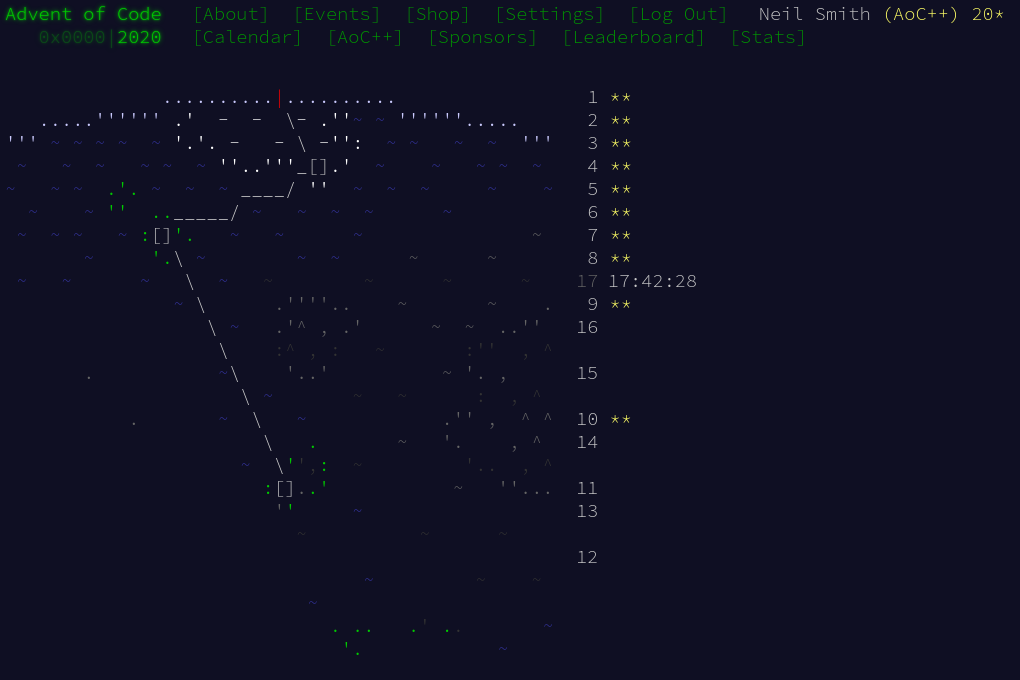December 17, 2020

# Advent of Code 2020 day 10

Dynamic programming done quickly.Day 10 is another example where the explanation is much longer than the code.

The first insight is to realise that the constraint that all adapters must be used, together with the fact that jolts can only increase, means that the adapter list should be sorted. At the same time, I include the source and the device in the list.

main :: IO ()
main =
let nums = map (read @Int) $lines numStrs let device = 3 + maximum nums let sortedNums = sort (0:device:nums) print$ part1 sortedNums
print $part2 sortedNums  ## Part 1 The only interesting part is the "trick" for bringing together adjacent pairs in the list: zip the list with the tail of itself. Once you have those pairs, you can filter if they have the desired gap, then count how many are left. part1 :: [Int] -> Int part1 nums = jolt1s * jolt3s where jolt1s = joltNs 1 nums jolt3s = joltNs 3 nums joltNs d ns = length$ filter diff $zip ns (tail ns) where diff (a, b) = (b - a) == d  ## Part 2 There are a couple of ways to approach this. One is to generate all the arrangements of adapters that lead from the source to the device, and count how many there are. As there are 100 adapters, that's 2100 ≈ 1030 possible different arrangements of including and excluding adapters. This approach will only work with memoisation. The other approach, the one I took, is dynamic programming. The solution is easy if I can just look up the answer. Imagine I have a table that shows, for each adapter, the number of arrangements that leads to that adapter. When I include a new adapter, I can look up the values for the next-smaller adapters, add them together, and that's the result for this adapter. If I walk up the adapter list in sorted order, adding to the table as I go, I always have the information to efficiently calculate result for the next adapter. The table is a Map of adapter value to number of ways of using it. I include a new adapter in the table by looking up all the earlier arrangements, adding their values, then inserting that new value into the table. includeAdapter arrangements adapter = M.insert adapter earlierCount arrangements where earlierArrangements = M.filterWithKey (\k _ -> (adapter - k) <= 3) arrangements earlierCount = M.foldl (+) 0 earlierArrangements  Building the table over the list of adapters is a fold over the list of adapters, starting with an initial table that says there's one way to use the source. Once done, I extract the value of the maximum key (the device). part2 :: [Int] -> Int part2 nums = snd$ M.findMax $foldl includeAdapter (M.singleton 0 1)$ tail nums